Connection between Frequency Response Data and Transfer Functions:

I. Some preliminaries on complex numbers.

1. Polar forms of complex numbers:

Let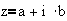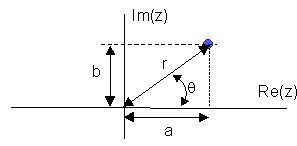then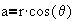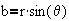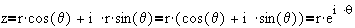where r is known as the magnitude of z, denoted |z|, and q is known as the argument of z, denoted arg(z)
Thus the polar form for z is given by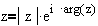where,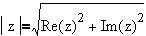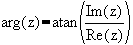2. Some properties of complex numbers:

Let conj(z) be the conjugate of z, e.g. conj(a+ i b)=a - i b
a)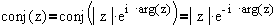b)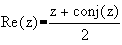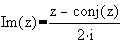3. Functions of complex numbers yield complex numbers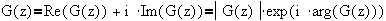4. A function of the conjugate is the conjugate of the function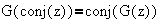Example: Let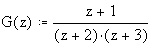then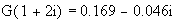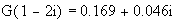Example: Let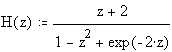then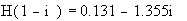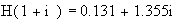II. Frequency Response of a stable Transfer Function

Suppose we are given a transfer function  G(s)  from u(s) to y(s), whose poles all have negative real parts. Using a sinusoidal input, u(t)=A sin(wt), the Laplace transform is given by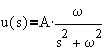The output, y(s) in the Laplace domain is given by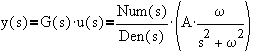For discussion purposes, we will use a second order process (with b,c>0)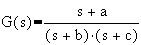then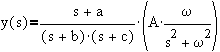After separation into partial fractions,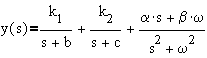Using the short cut method to determine a and b,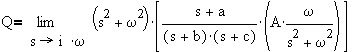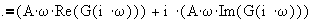which yields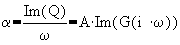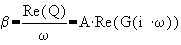Thus,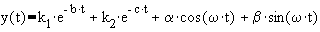As t goes large, the transients die out - leaving only the last two terms,
[Equation 1]: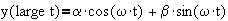Based on earlier discussions, this was put in the form,
[Equation 2]: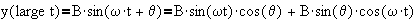Comparing equation 1 with equation 2,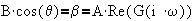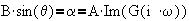After some more rearrangements,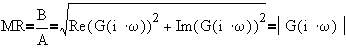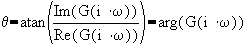This page is maintained by Tomas B. Co (tbco@mtu.edu). Last revised 2/3/1999.
Tomas B. Co
Associate Professor
Department of Chemical Engineering
Michigan Technological University
1400 Townsend Avenue
Houghton, MI 49931-1295